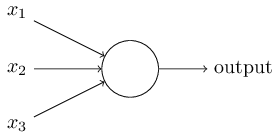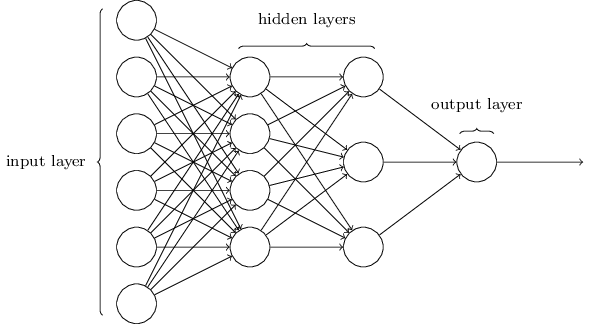《神经网络与深度学习》资源

## 一个人工神经元的结构• 若干个输入($x_1, x_2,… x_n$)，
• 只有一个输出值1. 将权重向量 $W = (w_1, w_2…w_n)$ 输入向量 $A = (a_1, a_2…a_n)^T$ 点乘得到的值加上偏置 $b$ 得到一个值 $z$。为了方便运算，我们也可以在输入向量 $A$ 后面添加一个1，这样就可以把偏置 $b$ 当做一个权重来看待

$z = w_1a_1 + w_2a_2 + … + w_na_n + b*1$

2. 对 $z$ 套用激活函数 $f$ 得到 $output = f(z)$

### S 型神经元

sigmoid函数表达式如下

$$f(z) = \frac{1}{1 + e^{-z}}$$

sigmoid函数有一个非常好的性质就是

$$f’(z) = f(z)(1 - f(z))$$## 神经网络的架构及常用符号• 输入层的输入即输出，没有权重、偏置和激活函数
• 隐藏层层数大于等于两层的神经网络称为深度神经网络
• 一般来讲，多分类问题中，有几个输出类别，输出层就有几个神经元
• 按照是否允许网络中存在反馈回路可以将网络分为前馈神经网络递归神经网络两类。相对简单，应用也最为广泛的是前馈神经网络。

## 神经网络的本质

$$output = f(z)$$

$$z = w_1a_1 + w_2a_2 + … + w_na_n + b*1$$

## 神经网络训练的本质

$$C(w, b) ≡ \frac{1}{2n}∑||y(x) - a||^2$$

## 梯度下降算法

$$∆C ≈ \frac{∂C}{∂v_1}∆v_1 + \frac{∂C}{∂v_2}∆v_2$$

$$∇C ≡ (\frac{∂C}{∂v_1}, \frac{∂C}{∂v_2})^T$$

$$∆C ≈ ∇C · ∆v$$

$$∆v = -η∇C$$

$$v→v′= v−η∇C$$

$$w_k→w_k′ =w_k−η\frac{∂C}{∂w_k}$$

$$b_l →b′_l =b_l −η\frac{∂C}{∂b_l}$$

### 普通梯度下降的问题

$$C(w, b) ≡ \frac{1}{2n}∑||y(x) - a||^2$$

### 随机梯度下降的思想

SGD的具体操作为，首先将全部训练数据打散（随机排序），然后将其分成一个个固定大小的片(batch)，然后遍历每个batch，对这个batch内的每一条数据求其 $∇C_x$，然后平均得到整体的 $∇C$ 来更新权重和偏置。

$$∇C ≈ \frac{1}{m}\Sigma_{j=1}^m∇C_{X_j}$$

$$w_k→w_k′= w_k−\frac{η}{m}\Sigma_j\frac{∂C_{X_j}}{∂w_k}$$

$$b_l→b_l′= b_l−\frac{η}{m}\Sigma_j\frac{∂C_{X_j}}{∂b_l}$$

### Batch, Iteration, Epoch的定义

• Batch：将训练数据随即打散然后按固定大小分割成的数据片叫做一个batch，其大小就是batch_size
• Iteration: 利用一个batch中的数据对权重和偏置的一次更新叫做一个iteration
• Epoch：当全部的batch都被便利了一遍，此时就叫一个epoch。

## 零散的知识点

• 随机梯度下降误差反向传递不适合直接用来训练深度神经网络
• 复杂的算法 ≤ 简单的算法 + 好的训练数据